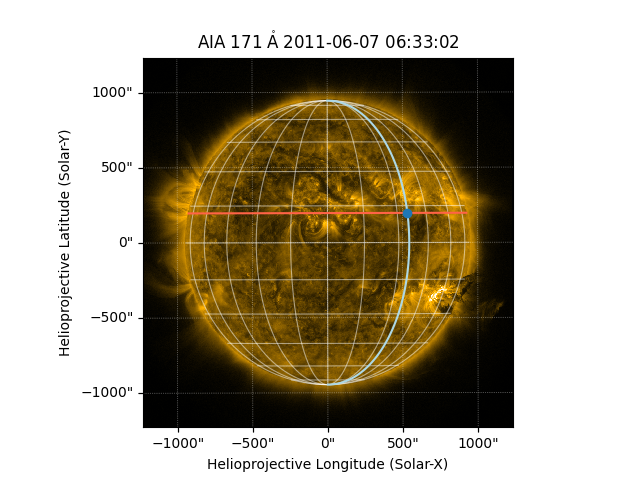# Drawing heliographic longitude and latitude lines¶

How to draw your own (Stonyhurst) longitude and latitude lines

```import numpy as np
import astropy.units as u
import matplotlib.pyplot as plt

from astropy.coordinates import SkyCoord

import sunpy.map
from sunpy.coordinates import frames
from sunpy.data.sample import AIA_171_IMAGE
```

The purpose of this example is to demonstrate the coordinate transformations that occur under the hood to show the heliographic grid lines of longitude latitude. We first create the Map using the sample data.

```aia = sunpy.map.Map(AIA_171_IMAGE)
```

Let’s first transform a single heliographic point coordinate.

```stonyhurst_center = SkyCoord(12 * u.deg, 12 * u.deg,
frame=frames.HeliographicStonyhurst)
```

Next we transform it into the coordinate frame of our map which is in helioprojective coordinates.

```hpc_stonyhurst_center = stonyhurst_center.transform_to(aia.coordinate_frame)
print(hpc_stonyhurst_center)
```

Out:

```<SkyCoord (Helioprojective: obstime=2011-06-07T06:33:02.770, rsun=696000000.0 m, observer=<HeliographicStonyhurst Coordinate (obstime=2011-06-07T06:33:02.770): (lon, lat, radius) in (deg, deg, m)
(-0.00406234, 0.04787238, 1.51846026e+11)>): (Tx, Ty, distance) in (arcsec, arcsec, km)
(193.09898111, 196.58816167, 1.51180424e+08)>
```

Now let’s transform two lines, one of longitude and one of of latitude. We define the coordinates as we did before and then transform them.

```num_points = 100
lat_value = 12 * u.deg
lon_value = 35 * u.deg
lon0 = SkyCoord(np.linspace(-80, 80, num_points) * u.deg,
np.ones(num_points) * lon_value, frame=frames.HeliographicStonyhurst)
lat0 = SkyCoord(np.ones(num_points) * lat_value,
np.linspace(-90, 90, num_points) * u.deg,
frame=frames.HeliographicStonyhurst)

hpc_lon0 = lon0.transform_to(aia.coordinate_frame)
hpc_lat0 = lat0.transform_to(aia.coordinate_frame)
```

Now let’s plot the results. We’ll overlay the autogenerated lon/lat grid as well for comparison.

```fig = plt.figure()
ax = plt.subplot(projection=aia)
aia.plot()
ax.plot_coord(hpc_lat0, color="C0")
ax.plot_coord(hpc_lon0, color="C0")
aia.draw_grid()
plt.show()
```Total running time of the script: ( 0 minutes 2.048 seconds)

Gallery generated by Sphinx-Gallery